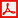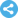International Journal of Mathematical Analysis and Applications
Manuscript Information

On a Problem Arising in a Two-Fluid Medium
International Journal of Mathematical Analysis and Applications
Vol.5 , No. 4, Publication Date: Dec. 24, 2018, Page: 95-100
5663 Views Since December 24, 2018, 469 Downloads Since Dec. 24, 2018Paper in PDF (273K)Follow on

Authors

  Sherzod Imomnazarov, Mathematics Department, Novosibirsk University, Novosibirsk, Russia.  Kholmatjon Imomnazarov, Mathematical Geophysics Department, Mathematics and Mathematical Geophysics Institute, Novosibirsk, Russia.  Abdulkhamid Kholmurodov, Physics and Mathematics Department, Karshi State University, Karshi, Uzbekistan.  Nasrutdin Dilmuradov, Physics and Mathematics Department, Karshi State University, Karshi, Uzbekistan.  Musajon Mamatkulov, Mechanics and Mathematics Department, National University of Uzbekistan, Tashkent, Uzbekistan.

Abstract

A nonlinear system of equations describing the dynamics of the motion of two-phase fluids is considered. An initial-boundary value problem for the systems of viscous two-fluid media with phase equilibrium with respect to pressure whose solution or some integral characteristic of this solution becomes infinite in a finite time (blow-up) is investigated. Using the method of test functions proposed by Pokhozhaev and Mitidieri, the influence of boundary and initial conditions on the appearance, time and rate of destruction of solutions to these problems is examined. The theorem on the blow-up of the solution to the initial-boundary value problem is proved. The lifetime of the solution is estimated.

Keywords

Two-Speed Hydrodynamics, Mineralization, Porous Medium, Initial-Boundary Value Problem, Destruction in a Finite Time, Blow-Up

Reference

  Kadet V. v., Koryuzlov A. S. Effective viscosity of mineralizaed water when flowing in a porous medium // Theory and Experiment, Theoretical Grounds of Chemical Technologies, 2008, v. 42, No. 5. pp. 703-708 (in Russian).  Dorovsky V. N., Podberezhnyy M., Nefedkin Y. Stoneley attenuation length and pore fluid salinity // Russian Geology and Geophysics. 2011. v. 52. No. 2. pp. 250-258 (in Russian).  Landau L. D., Lifshits Ye. M. Fluid Mechanics, Pergamon Press, New York, 1989.  Dorovsky V. N. Mathematical models of two-velocity media, Mathematical and Computer Modelling, 1995, v. 21, No. 7, pp. 17-28.  DorovskyV. N., Perepechko Yu. V. Mathematical models of two-velocity media. Part II, Mathematical and Computer Modelling, 1996, v. 24, No. 10, pp. 69-80.  Dorovsky V. N., Perepechko Yu. V. Hydrodynamic model of the solution in fractured porous media // Russian Geology and Geophysics. 1996. No. 9. pp. 123-134 (in Russian).  Dorovsky V. N. The hydrodynamics of particles suspended in a melt with the self-consistent concentration field of an admixture, I, Computers and Mathematics with Applications. 1998. v. 35, No. 11. pp. 27-37.  Pokhozhaev S. I. Riemann quasi-invariants // Sb. Math., 2011, v. 202, No. 6, pp. 887-907.  Pokhozhaev S. I. Weighted Identities for the Solutions of Generalized Korteweg-de Vries Equations // Math. Notes, 2011, v. 89, No. 3, pp. 382-396.  Pokhozaev S. I. On the absence of global solutions of the Korteweg-de Vries equation // Journal of Mathematical Sciences, 2013, v. 190, No. 1, pp. 147-156.  Mitidieri E., Pokhozhaev S. I. A priori estimates and blow-up of solutions to nonlinear partial differential equations and inequalities // Proc. Steklov Inst. Math., 2001, v. 234, pp. 1-362.  Galaktionov V. A., Mitidieri E., Pokhozhaev S. I. On global solutions and blow-up for Kuramoto-Sivashinsky-type models, and well-posed Burnett equations // Nonlinear Anal., 2009, v. 70, No. 8 pp. 2930-2952.  Pokhozhaev S. I. On the nonexistence of global solutions for some initial-boundary value problems for the Korteweg-de Vries equation // Differ. Equ., 2011, v. 47, No. 4, pp. 488-493.  Yushkov E. V. On destruction of a solution in systems of the Korteweg — de Vries type // TMF, 2012, v. 173, No. 2, pp. 197-206 (in Russian).  Dobrokhotov S. Yu., Shafarevich A. I. On the behavior on infinity of the velocity field of incompressible fluid // Mekhanika dzidkosti i gaza, 1996, v. 31, No. 4, pp. 38-42 (in Russian).  Yushkov E. V. On the blow-up of solutions of equations of hydrodynamic type under special boundary conditions // Differential Equations. 2012. Vol. 48, No. 9. pp. 1212-1218.  Lai S., Wu Y. Global solutions and blow-up phenomena to a shallow water equation // J. Differ. Equ., 2010, v. 249, No. 3, pp. 693-706.  Perepechko Yu. V. Equations for the flow of mineralized fluid in a porous medium // Proceedings of the scientific. conf. KarshiSU "Actual questions of analysis", April 22-23, 2016, Karshi, pp. 162-164 (in Russian).  Godunov S. K., Romensky E. I. Elements of continuum mechanics and conservation laws, M.: Scientific book, 1998 (in Russian).  de Groot S. R., Marur P. Nonequilibrium thermodynamics. Amsterdam: NorthHolland, 1962. 510 p.